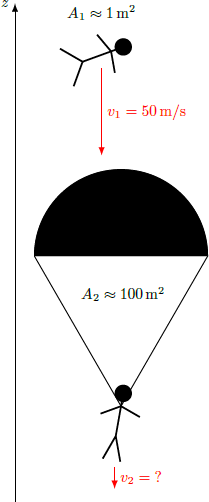# Opening a parachuteWithout opening his parachute, a skydiver reaches a fall velocity of $v_1 = 50\text { m/s}.$ When he does open the parachute, he's braked by additional air resistance. After a while, he finally arrives at the ground.

At what fall velocity $v_2$ does he reach the ground?

Details and Assumptions:

• The air frictional force $F_f = F_f (\rho, A, v)$ depends only on the density of the air $\rho ,$ the cross-sectional area of the skydiver $A,$ and the velocity $v.$ Neglect the effect that the shape of the falling object has on the air frictional force.
• The cross-sectional area without the parachute is $A_1 \approx 1\text { m}^2$ and that with the parachute is $A_2 \approx 100\text{ m}^2.$
• The density of the air is constant.
×